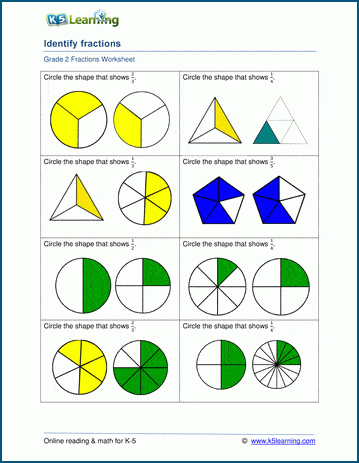# Identifying fractions

## Identifying basic fractions worksheets

Students need to learn to recognize basic fractions such as 1/2, 1/3, etc.  These worksheets represent simple fractions with shapes divided into equal parts; students select which shape equates to the given fraction.## More fractions worksheets

Explore all of our fractions worksheets, from dividing shapes into "equal parts" to multiplying and dividing improper fractions and mixed numbers.

## What is K5?

K5 Learning offers reading and math worksheets, workbooks and an online reading and math program for kids in kindergarten to grade 5.  We help your children build good study habits and excel in school.